# Case Study Undefined Variables CS 5010 Program Design

• Slides: 30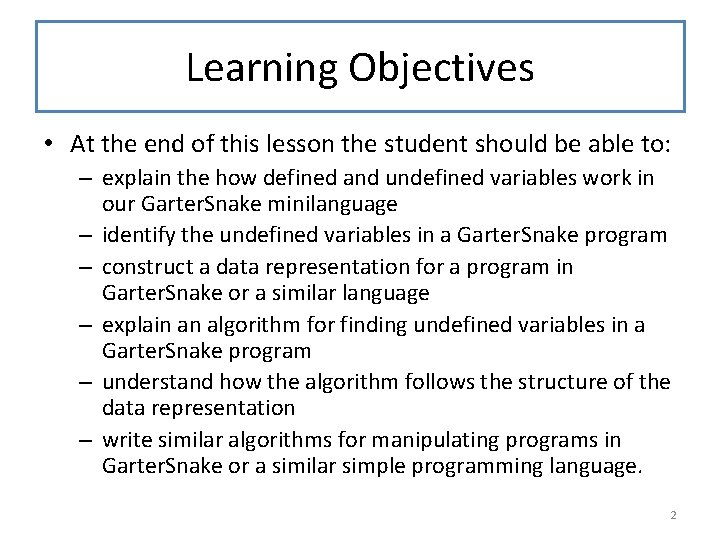Learning Objectives • At the end of this lesson the student should be able to: – explain the how defined and undefined variables work in our Garter. Snake minilanguage – identify the undefined variables in a Garter. Snake program – construct a data representation for a program in Garter. Snake or a similar language – explain an algorithm for finding undefined variables in a Garter. Snake program – understand how the algorithm follows the structure of the data representation – write similar algorithms for manipulating programs in Garter. Snake or a similar simple programming language. 2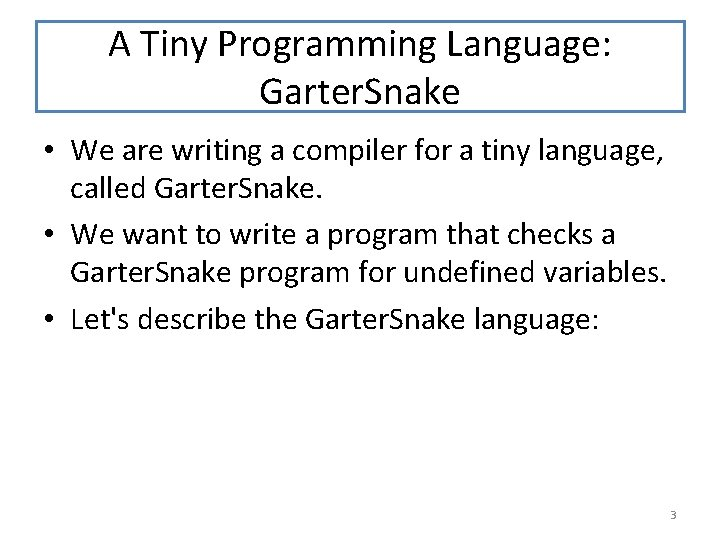A Tiny Programming Language: Garter. Snake • We are writing a compiler for a tiny language, called Garter. Snake. • We want to write a program that checks a Garter. Snake program for undefined variables. • Let's describe the Garter. Snake language: 3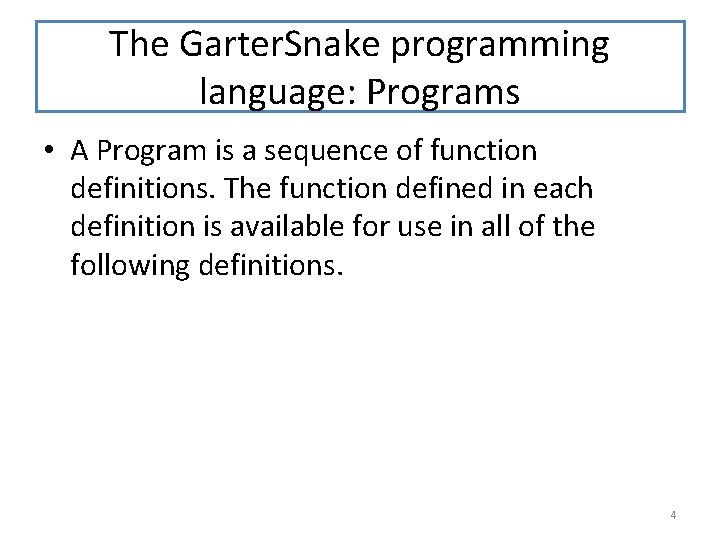The Garter. Snake programming language: Programs • A Program is a sequence of function definitions. The function defined in each definition is available for use in all of the following definitions. 4Example: A Garter. Snake program def f 1(x): f 1(x) ; f 1 is available in the body of f 1 def f 2 (x, y): f 1(y) ; f 1 is available in the body of f 2 ; spaces are ignored def f 3 (x, z): f 1(f 2(z, f 1)) ; f 1 and f 2 are available in the body of f 3 ; you can pass a function as an argument def f 4 (x, z): x(z, z) ; you can call an argument as a function 5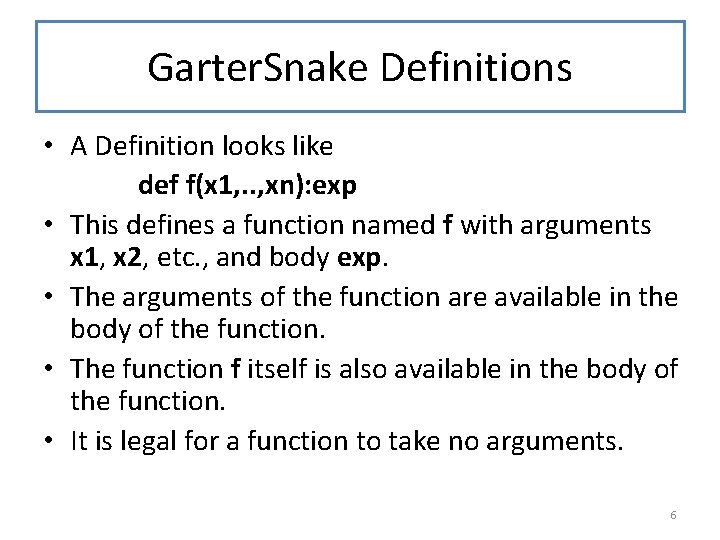Garter. Snake Definitions • A Definition looks like def f(x 1, . . , xn): exp • This defines a function named f with arguments x 1, x 2, etc. , and body exp. • The arguments of the function are available in the body of the function. • The function f itself is also available in the body of the function. • It is legal for a function to take no arguments. 6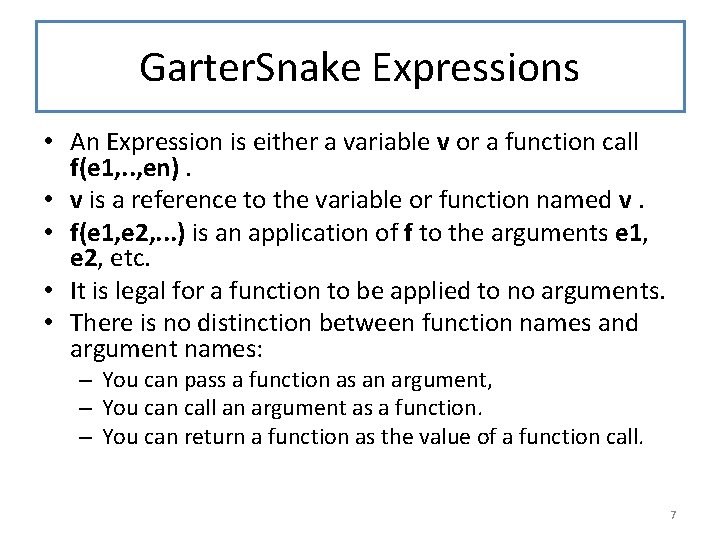Garter. Snake Expressions • An Expression is either a variable v or a function call f(e 1, . . , en). • v is a reference to the variable or function named v. • f(e 1, e 2, . . . ) is an application of f to the arguments e 1, e 2, etc. • It is legal for a function to be applied to no arguments. • There is no distinction between function names and argument names: – You can pass a function as an argument, – You can call an argument as a function. – You can return a function as the value of a function call. 7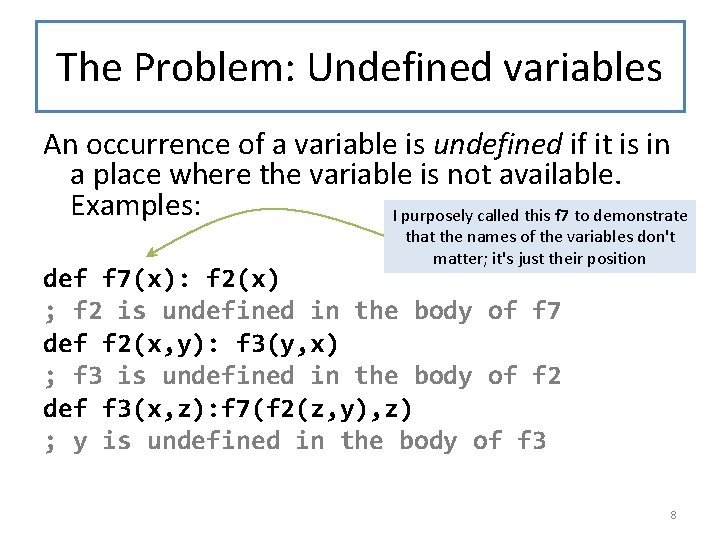The Problem: Undefined variables An occurrence of a variable is undefined if it is in a place where the variable is not available. Examples: I purposely called this f 7 to demonstrate that the names of the variables don't matter; it's just their position def f 7(x): f 2(x) ; f 2 is undefined in the body of f 7 def f 2(x, y): f 3(y, x) ; f 3 is undefined in the body of f 2 def f 3(x, z): f 7(f 2(z, y), z) ; y is undefined in the body of f 3 8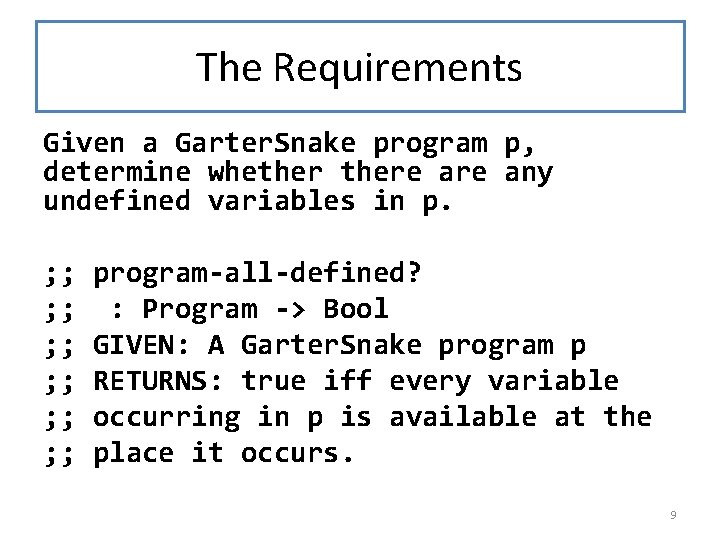The Requirements Given a Garter. Snake program p, determine whethere any undefined variables in p. ; ; ; program-all-defined? : Program -> Bool GIVEN: A Garter. Snake program p RETURNS: true iff every variable occurring in p is available at the place it occurs. 9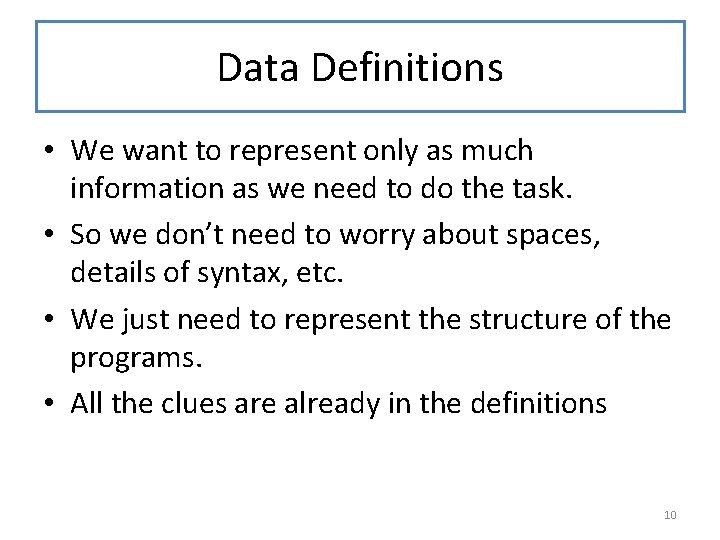Data Definitions • We want to represent only as much information as we need to do the task. • So we don’t need to worry about spaces, details of syntax, etc. • We just need to represent the structure of the programs. • All the clues are already in the definitions 10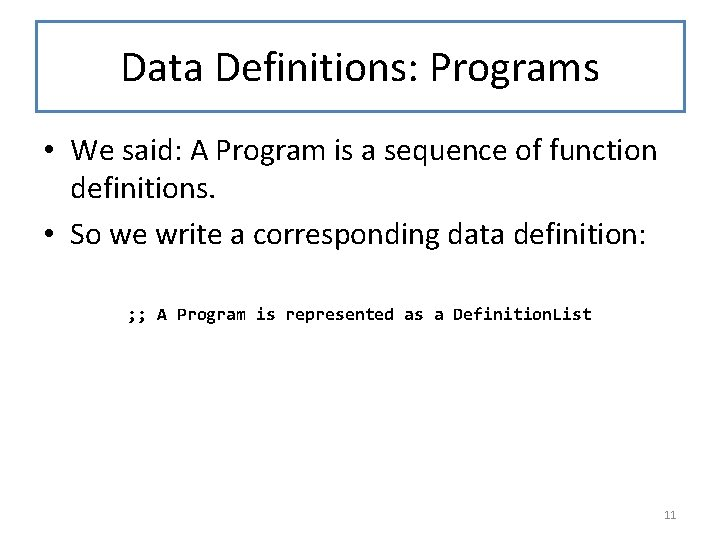Data Definitions: Programs • We said: A Program is a sequence of function definitions. • So we write a corresponding data definition: ; ; A Program is represented as a Definition. List 11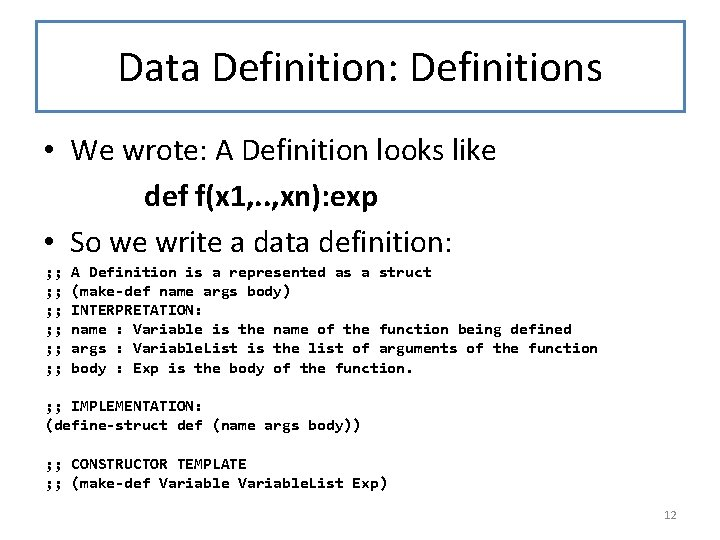Data Definition: Definitions • We wrote: A Definition looks like def f(x 1, . . , xn): exp • So we write a data definition: ; ; ; A Definition is a represented as a struct (make-def name args body) INTERPRETATION: name : Variable is the name of the function being defined args : Variable. List is the list of arguments of the function body : Exp is the body of the function. ; ; IMPLEMENTATION: (define-struct def (name args body)) ; ; CONSTRUCTOR TEMPLATE ; ; (make-def Variable. List Exp) 12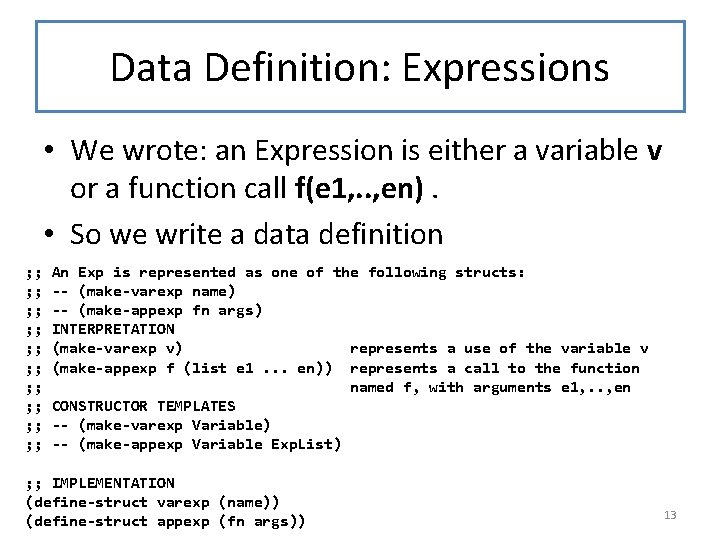Data Definition: Expressions • We wrote: an Expression is either a variable v or a function call f(e 1, . . , en). • So we write a data definition ; ; ; ; ; An Exp is represented as one of the following structs: -- (make-varexp name) -- (make-appexp fn args) INTERPRETATION (make-varexp v) represents a use of the variable v (make-appexp f (list e 1. . . en)) represents a call to the function named f, with arguments e 1, . . , en CONSTRUCTOR TEMPLATES -- (make-varexp Variable) -- (make-appexp Variable Exp. List) ; ; IMPLEMENTATION (define-struct varexp (name)) (define-struct appexp (fn args)) 13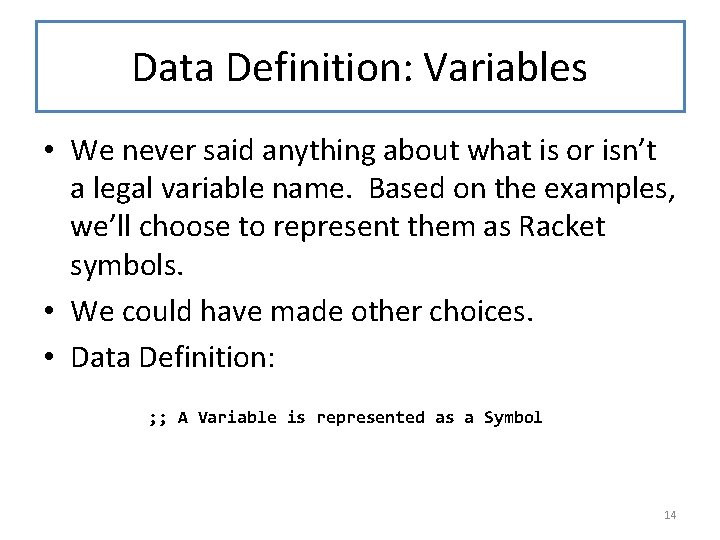Data Definition: Variables • We never said anything about what is or isn’t a legal variable name. Based on the examples, we’ll choose to represent them as Racket symbols. • We could have made other choices. • Data Definition: ; ; A Variable is represented as a Symbol 14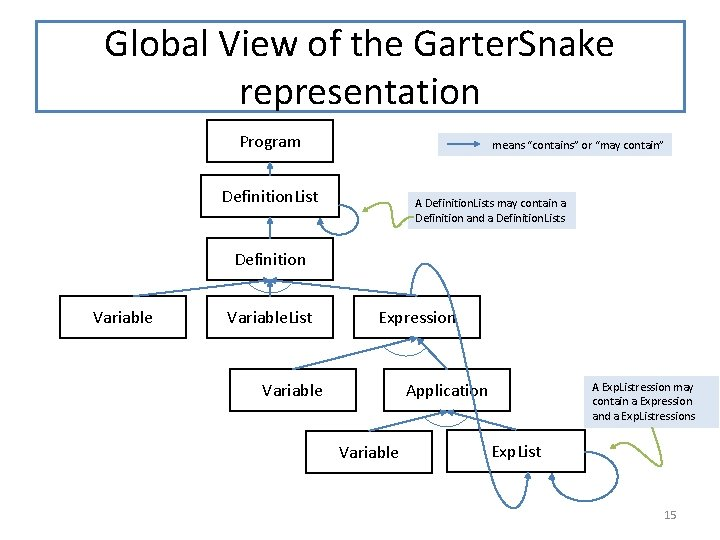Global View of the Garter. Snake representation Program means “contains” or “may contain” Definition. List A Definition. Lists may contain a Definition and a Definition. Lists Definition Variable. List Expression Variable Application Variable A Exp. Listression may contain a Expression and a Exp. Listressions Exp. List 15Observer Templates ; ; pgm-fn : Program -> ? ? #; (define (pgm-fn p) (deflist-fn p)) ; ; def-fn : Definition -> ? ? #; (define (def-fn d) (. . . (def-name d) (def-args d) (def-body d))) In Racket, #; marks the next Sexpression as a comment. So this definition is actually a comment. This is handy for templates. ; ; exp-fn : Exp -> ? ? #; (define (exp-fn e) (cond [(varexp? e) (. . . (varexp-name e))] [(appexp? e) (. . . (appexp-fn e) (explist-fn (appexp-args e)))])) ; ; We omit the List. Of-* templates because they are standard and you should know ; ; them by heart already. 16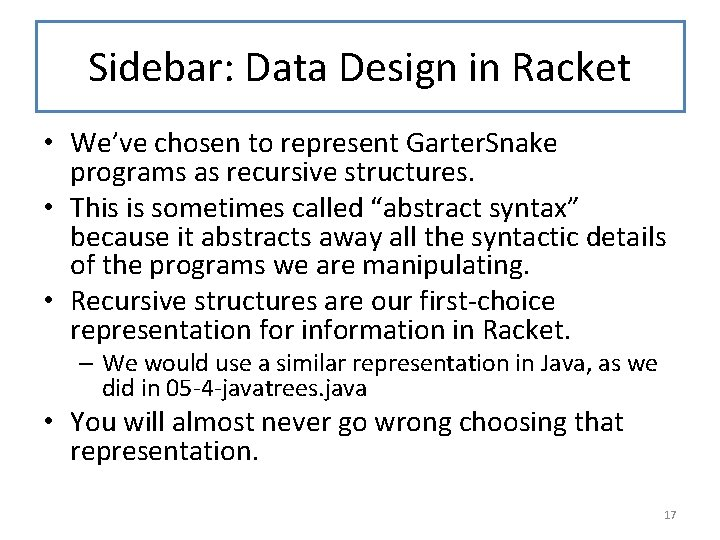Sidebar: Data Design in Racket • We’ve chosen to represent Garter. Snake programs as recursive structures. • This is sometimes called “abstract syntax” because it abstracts away all the syntactic details of the programs we are manipulating. • Recursive structures are our first-choice representation for information in Racket. – We would use a similar representation in Java, as we did in 05 -4 -javatrees. java • You will almost never go wrong choosing that representation. 17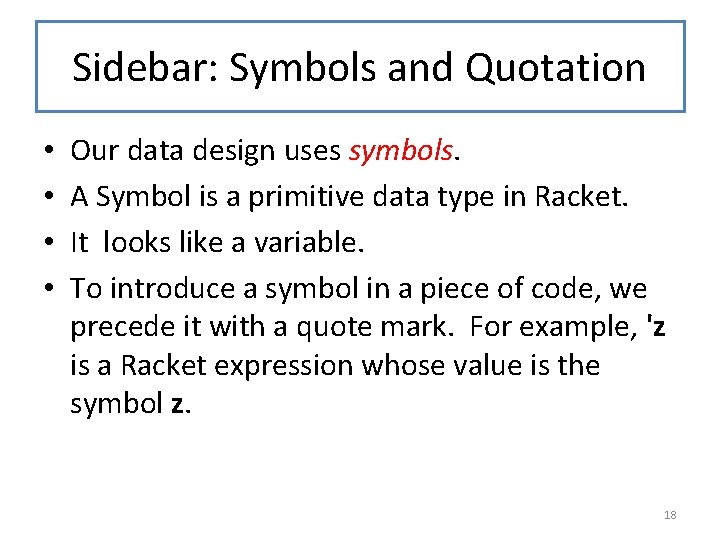Sidebar: Symbols and Quotation • • Our data design uses symbols. A Symbol is a primitive data type in Racket. It looks like a variable. To introduce a symbol in a piece of code, we precede it with a quote mark. For example, 'z is a Racket expression whose value is the symbol z. 18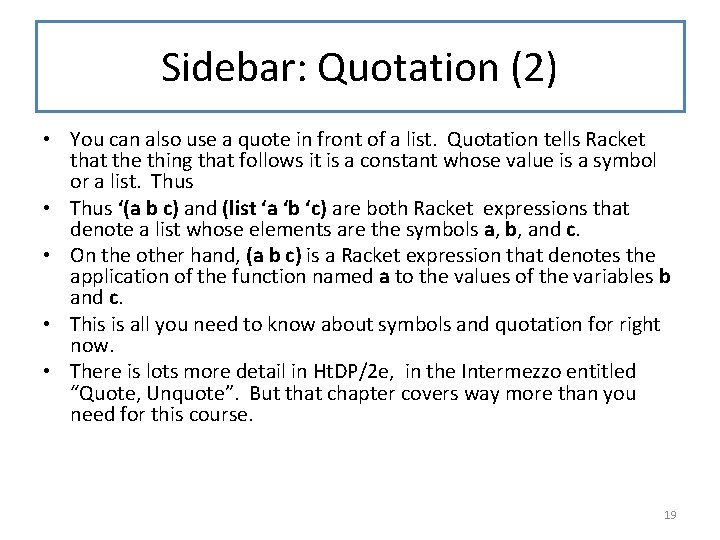Sidebar: Quotation (2) • You can also use a quote in front of a list. Quotation tells Racket that the thing that follows it is a constant whose value is a symbol or a list. Thus • Thus ‘(a b c) and (list ‘a ‘b ‘c) are both Racket expressions that denote a list whose elements are the symbols a, b, and c. • On the other hand, (a b c) is a Racket expression that denotes the application of the function named a to the values of the variables b and c. • This is all you need to know about symbols and quotation for right now. • There is lots more detail in Ht. DP/2 e, in the Intermezzo entitled “Quote, Unquote”. But that chapter covers way more than you need for this course. 19Data Design: Example EXAMPLE: Now that we’ve briefly explained about def f 1(x): f 1(x) symbols and quotation, we can give an def f 2(x, y): f 1(y) example of the representation of a def f 3(x, y, z): f 1(f 2(z, y), z) Garter. Snake program is represented by (list (make-def 'f 1 (list 'x) (make-appexp 'f 1 (list (make-varexp 'x)))) (make-def 'f 2 (list 'x 'y) (make-appexp 'f 1 (list (make-varexp 'y)))) (make-def 'f 3 (list 'x 'y 'z) (make-appexp 'f 1 (list (make-appexp 'f 2 (list (make-varexp 'z) (make-varexp 'y))) (make-varexp 'z))))))) 20System Design (1) ; ; ; ; We'll need to recur on the list structure of programs. When we analyze a definition, what information do we need to carry forward? Let's look at an example. We'll annotate each definition with a list of the variables available in its body. #| def f 1(x): f 1(x) def f 2(u, y): f 1(y) def f 3(x, z): f 1(f 2(z, f 1)) def f 4(x, z): x(z, z) body. |# ; ; f 1 and x are available in the body. f 1, f 2, u, and y, are available in the body. f 1, f 2, f 3, x, and z are available in the body. f 1, f 2, f 3, f 4, x, and z are available in the ; ; In each case, the variables available in the body are the names of ; ; the functions defined _before_ the current function, plus the names ; ; of the current function and its arguments. 21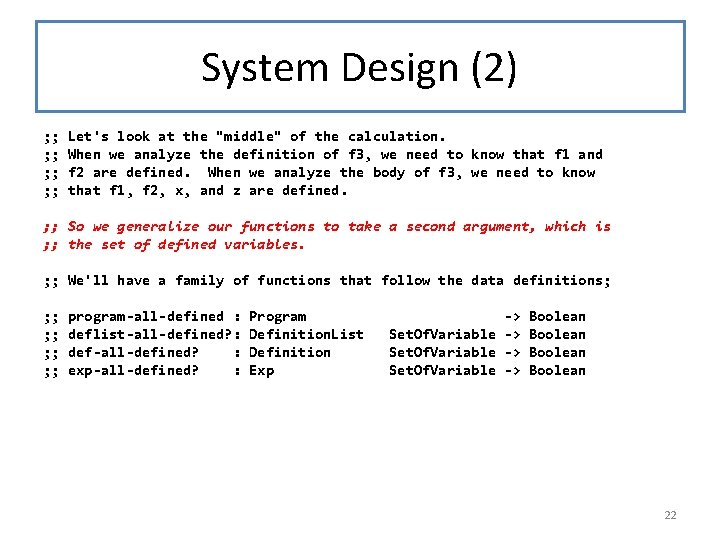System Design (2) ; ; ; ; Let's look at the "middle" of the calculation. When we analyze the definition of f 3, we need to know that f 1 and f 2 are defined. When we analyze the body of f 3, we need to know that f 1, f 2, x, and z are defined. ; ; So we generalize our functions to take a second argument, which is ; ; the set of defined variables. ; ; We'll have a family of functions that follow the data definitions; ; ; ; ; program-all-defined : deflist-all-defined? : def-all-defined? : exp-all-defined? : Program Definition. List Definition Exp -> Set. Of. Variable -> Boolean 22deflist-all-defined? ; ; ; ; ; deflist-all-defined? : Definition. List Set. Of. Variable -> Boolean GIVEN: a list of definitions 'defs' from some program p and a set of variables 'vars' WHERE: vars is the set of variables available at the start of defs in p. RETURNS: true iff there are no undefined variables in defs. You can’t tell if a EXAMPLES: See examples above (slide 8) variable is STRATEGY: Use template for Definition. List on defs. The names available in (rest defs) are those in vars, plus the variable undefined unless defined in (first defs). (define (deflist-all-defined? defs vars) (cond [(null? defs) true] [else (and (def-all-defined? (first defs) vars) (deflist-all-defined? (rest defs) (set-cons (def-name (first defs)) vars)))])) you know something about the program it occurs in! The WHERE invariant captures this information. Don’t say “see examples above” or “see tests below” unless there really are such examples or tests. 23def-all-defined? ; ; ; ; ; def-all-defined? : Definition Set. Of. Variable -> Boolean GIVEN: A definition 'def' from some program p and a set of variables 'vars' WHERE: vars is the set of variables available at the start of def in p. RETURNS: true if there are no undefined variables in the body of def. The available variables in the body are the ones in def, plus the name and arguments of the definition. EXAMPLES: See examples above (slide 8) STRATEGY: Use template for Definition on def (define (def-all-defined? def vars) (exp-all-defined? (def-body def) (set-cons (def-name def) (set-union (def-args def) vars)))) 24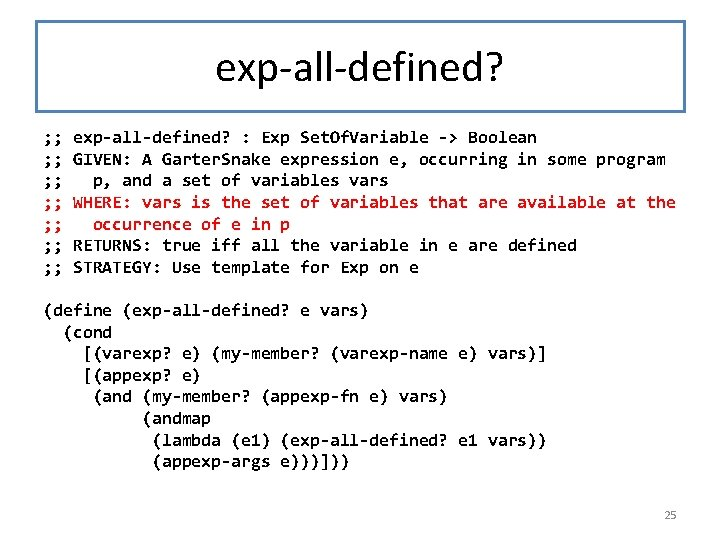exp-all-defined? ; ; ; ; exp-all-defined? : Exp Set. Of. Variable -> Boolean GIVEN: A Garter. Snake expression e, occurring in some program p, and a set of variables vars WHERE: vars is the set of variables that are available at the occurrence of e in p RETURNS: true iff all the variable in e are defined STRATEGY: Use template for Exp on e (define (exp-all-defined? e vars) (cond [(varexp? e) (my-member? (varexp-name e) vars)] [(appexp? e) (and (my-member? (appexp-fn e) vars) (andmap (lambda (e 1) (exp-all-defined? e 1 vars)) (appexp-args e)))])) 25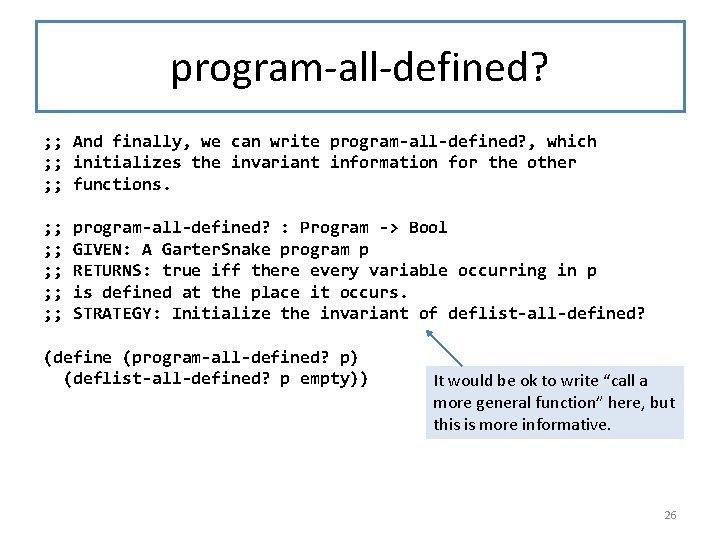program-all-defined? ; ; And finally, we can write program-all-defined? , which ; ; initializes the invariant information for the other ; ; functions. ; ; ; ; ; program-all-defined? : Program -> Bool GIVEN: A Garter. Snake program p RETURNS: true iff there every variable occurring in p is defined at the place it occurs. STRATEGY: Initialize the invariant of deflist-all-defined? (define (program-all-defined? p) (deflist-all-defined? p empty)) It would be ok to write “call a more general function” here, but this is more informative. 26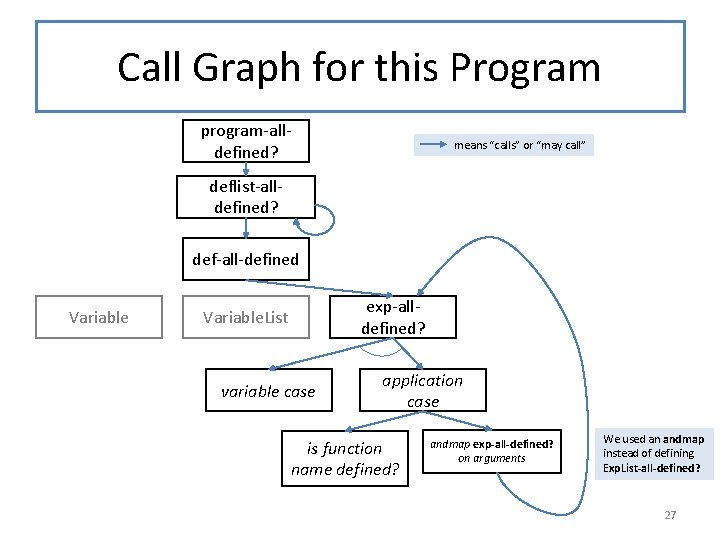Call Graph for this Program program-alldefined? means “calls” or “may call” deflist-alldefined? def-all-defined Variable exp-alldefined? Variable. List variable case application case is function name defined? andmap exp-all-defined? on arguments We used an andmap instead of defining Exp. List-all-defined? 27See how the call graph follows the structure of the data! Program means “contains” or “may contain” Definition. List A Definition. Lists may contain a Definition and a Definition. Lists Definition Variable. List Expression Variable Application Variable A Exp. Listression may contain a Expression and a Exp. Listressions Exp. Listression 28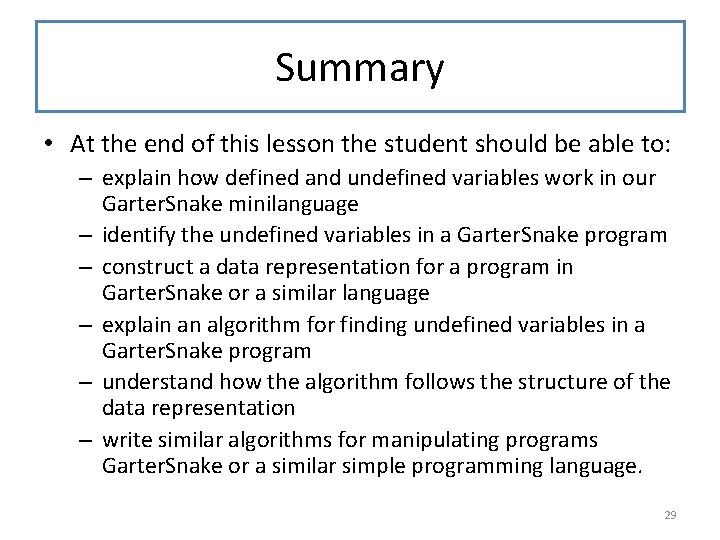Summary • At the end of this lesson the student should be able to: – explain how defined and undefined variables work in our Garter. Snake minilanguage – identify the undefined variables in a Garter. Snake program – construct a data representation for a program in Garter. Snake or a similar language – explain an algorithm for finding undefined variables in a Garter. Snake program – understand how the algorithm follows the structure of the data representation – write similar algorithms for manipulating programs Garter. Snake or a similar simple programming language. 29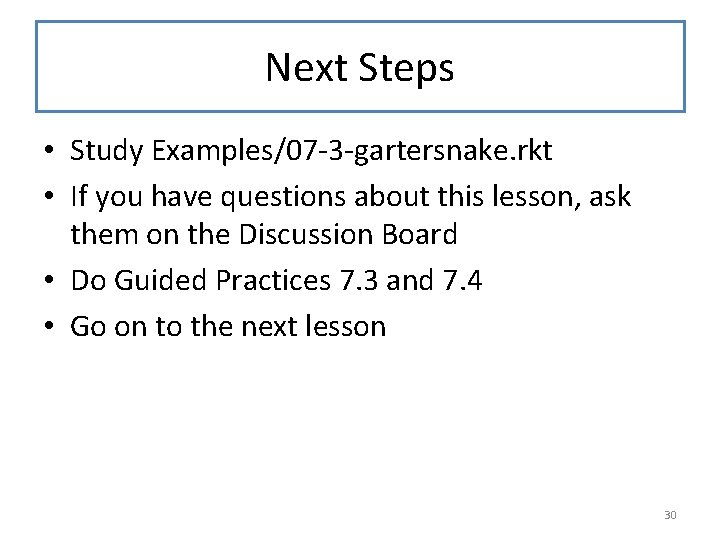Next Steps • Study Examples/07 -3 -gartersnake. rkt • If you have questions about this lesson, ask them on the Discussion Board • Do Guided Practices 7. 3 and 7. 4 • Go on to the next lesson 30